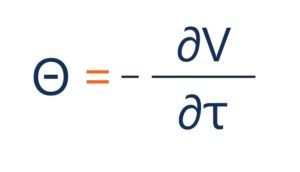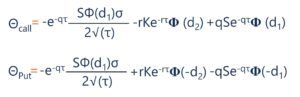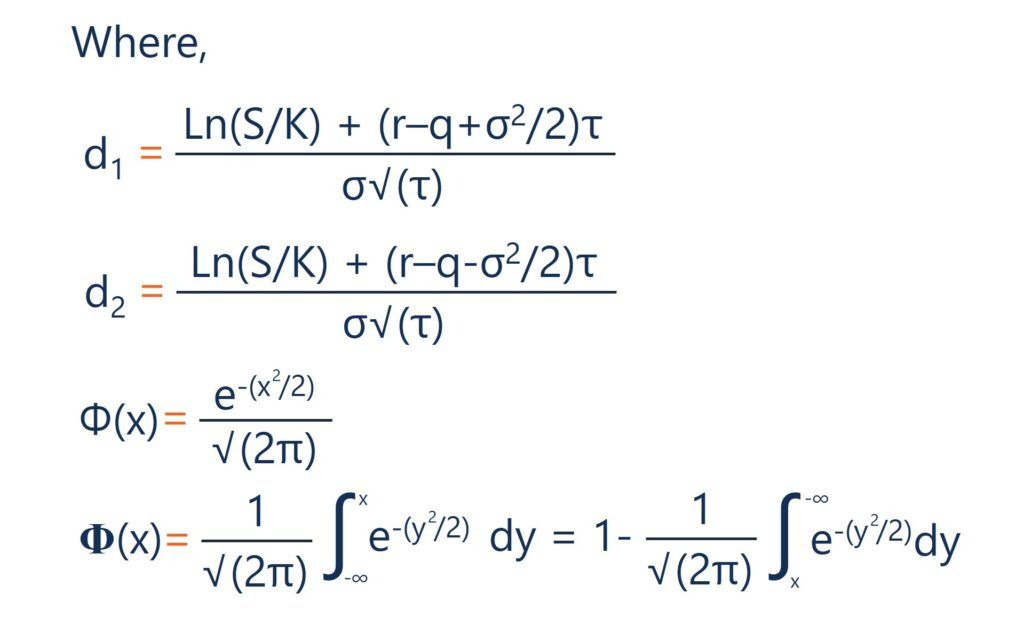# Theta (Θ)

A sensitivity measure of an option's value to time

## What is Theta?

Theta is a sensitivity measurement used in assessing derivatives. It is one of the measures denoted by a Greek letter. The series of risk and sensitivity measurements denoted by Greek letters are aptly named the Greeks. Theta measures the value of a derivative in relation to the time left before the expiration date. As an option gets closer to the expiration date, it will lose value priced into the extrinsic value. Theta can be thought of as the rate of decline in the value of an option as time passes.### Quick Summary of Points

• Theta is a sensitivity measure used in assessing an option’s value in relation to the time until expiration.
• The metric can be thought of as the rate of decline in the value of an option as time passes.
• Theta can be used to assess how much the underlying asset must change in value to offset the value lost to time decay.

### How to Interpret Theta?

To understand theta, it is important to first understand the difference between the intrinsic and extrinsic value of an option. Together, the extrinsic and intrinsic value make up the total value or premium of an option. The intrinsic value only measures the profit of the option based on the strike price and market price. One way to think about the intrinsic value is that if the option were to expire today, the premium consists only of this intrinsic value (strike price – market price). The extrinsic value, on the other hand, measures the part of the premium not defined by the intrinsic value. The extrinsic value is the value of being able to hold the option and the opportunity for the option to gain value as the underlying asset moves in price. The closer an option is to expiration, the smaller the extrinsic value becomes.

We now know that the further away from expiration the option is, the higher the extrinsic value. The closer to expiration the option is, the smaller the extrinsic value. At the expiration date, the extrinsic value is 0, and the entire premium consists of the intrinsic value assuming the option is in the money. Theta is a sensitivity measure that determines the decline in this extrinsic value of the option over time.

The calculation of theta is expressed as a yearly value; however, the figure is often divided by the number of days in a year to arrive at a daily rate. The daily rate is the amount the value will drop by. A theta of -0.20 means that the price of an option would fall by \$0.20 per day. In two days time, the price of the option would’ve fallen by \$0.40. However, it is important to note that theta is not constant over the lifetime of the option. As the option gets closer to the expiration date, theta increases and the value lost to time decay picks up.

### What is Theta Used for in Options?

As stated above, theta is used to gauge the sensitivity of the option value to time. It can be used to see how much value an option loses on a daily basis, and how much the underlying asset will need to change to offset the loss in according to theta. The measurement is not used by traders as often as other measures such as delta. However, buyers may still want to know what to expect in terms of time changes to an option. Option writers who take a short position may also consider theta since they will benefit from the time decay.

### How is Theta Calculated?

When the value of a long position of an option falls, the closer it is to maturity, which shows an inverse relationship. For long positions on an option, theta is generally negative, and for short positions on an option, theta is generally positive. Theta can be shown in the following general form:Where:

• ∂ – the first derivative
• V – the option’s price (theoretical value)
• τ – the option’s time to maturity

Under the Black-Scholes model, the calculation for theta is given by:Where:

• S – the stock price
• K – the strike price
• r – the risk-free rate
• q – the annual dividend yield
• τ – time until expiration
• σ – the volatility